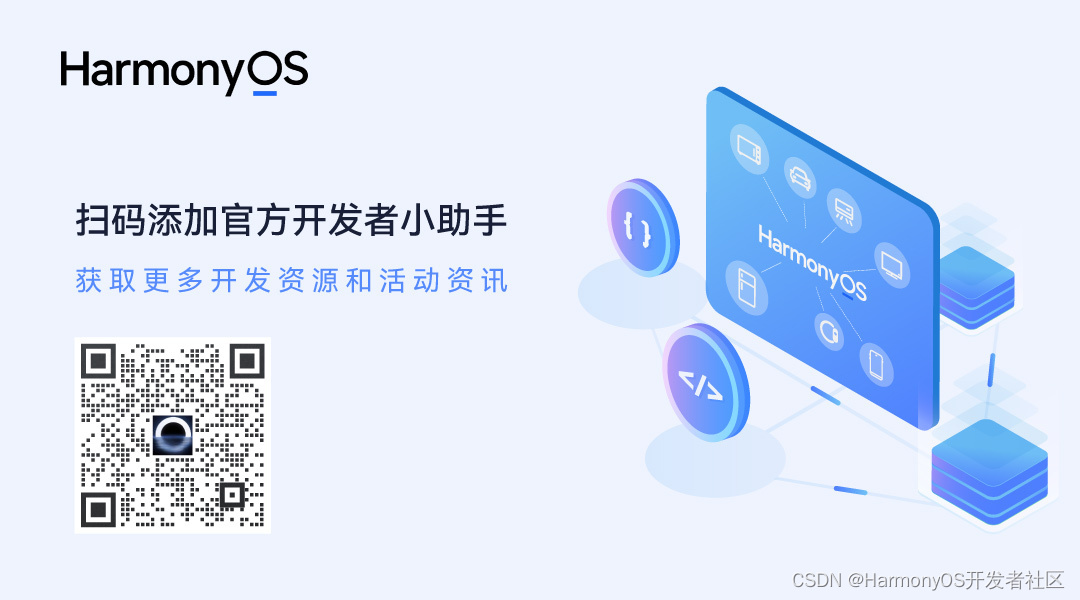> 文档中心 > 基于ArkUI开发框架，图片马赛克处理的实现

# 基于ArkUI开发框架，图片马赛克处理的实现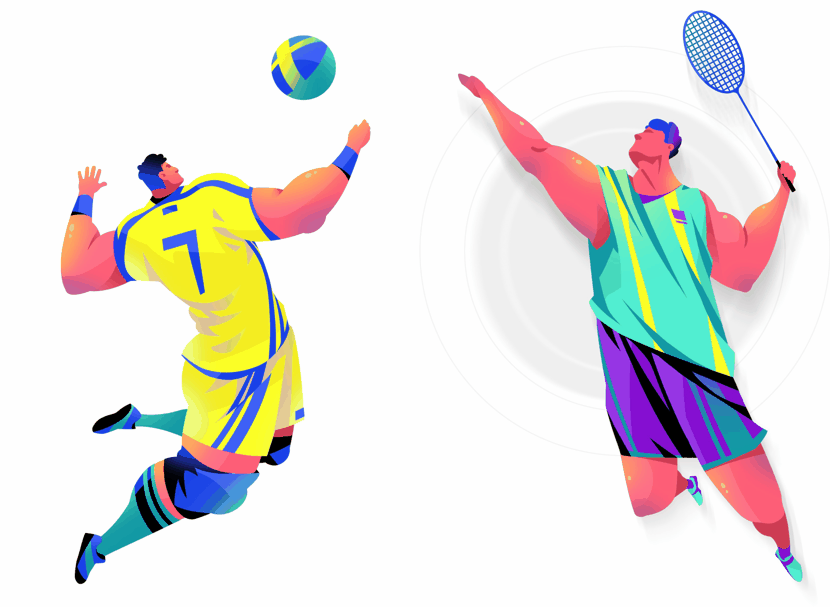1. 像素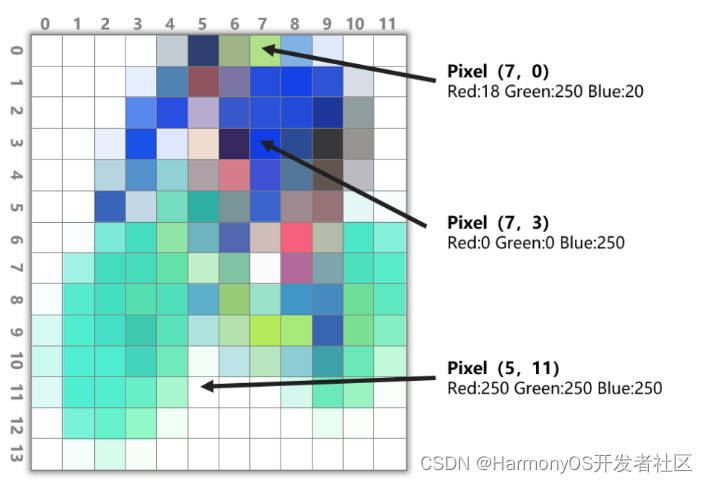图1 像素点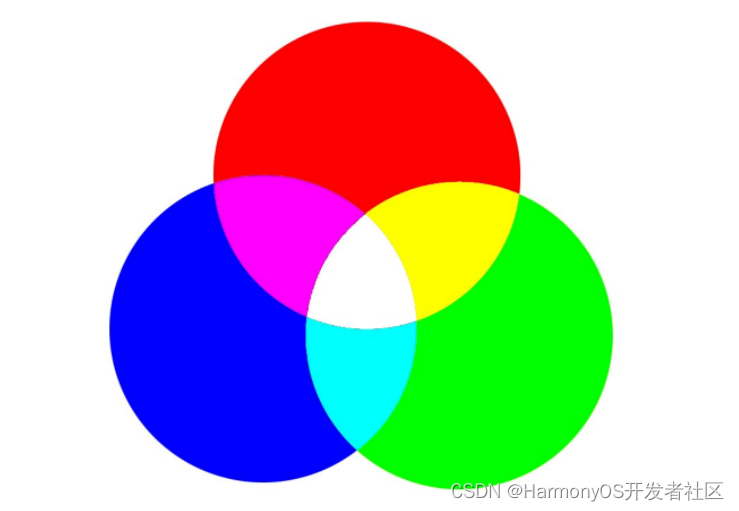图2 光学三原色

2. 分辨率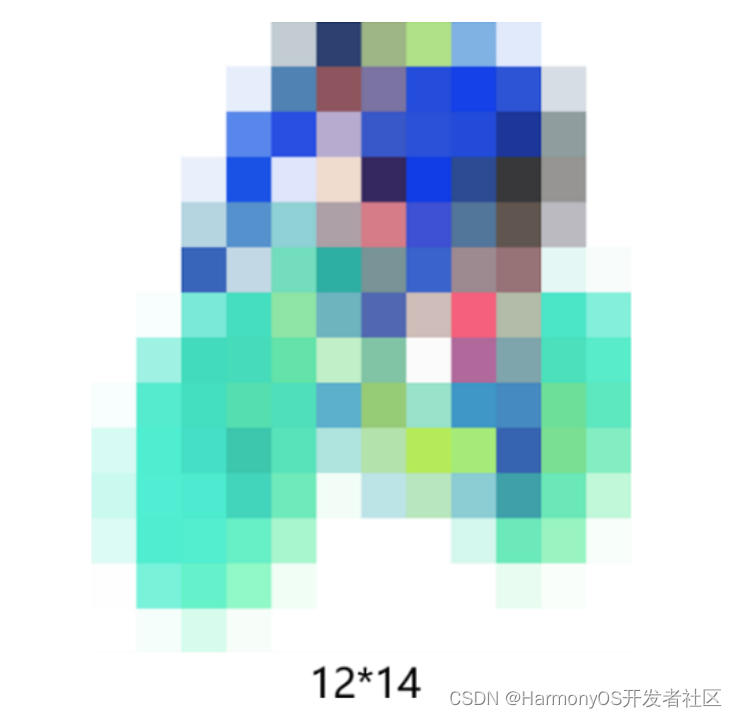图3 低分辨率图片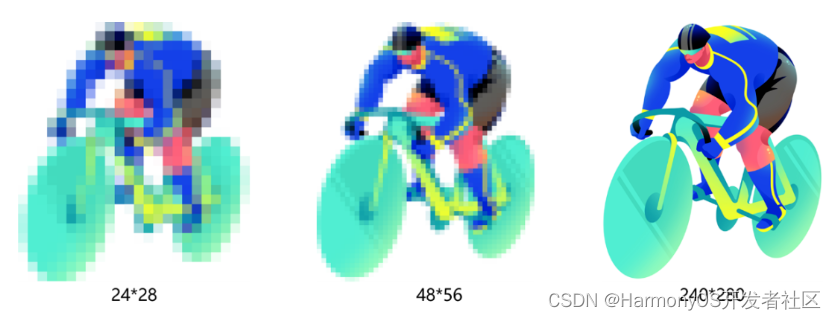图4 分辨率的变化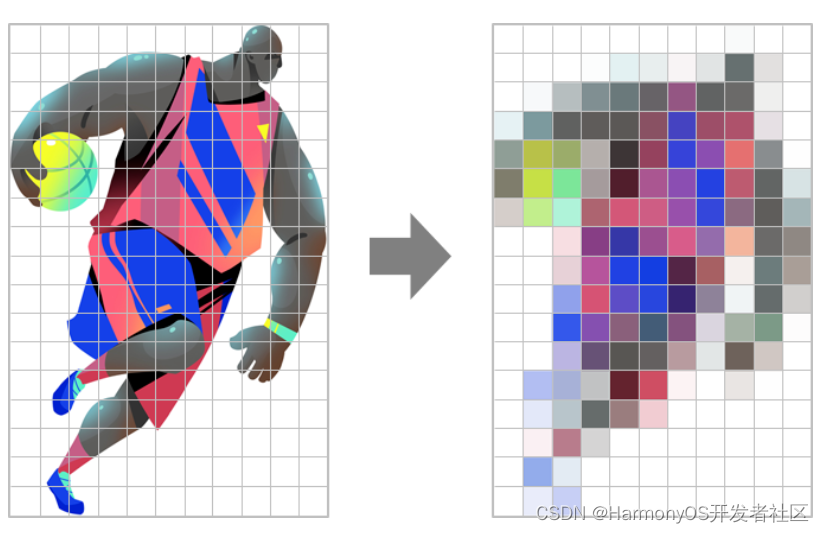图5 马赛克原理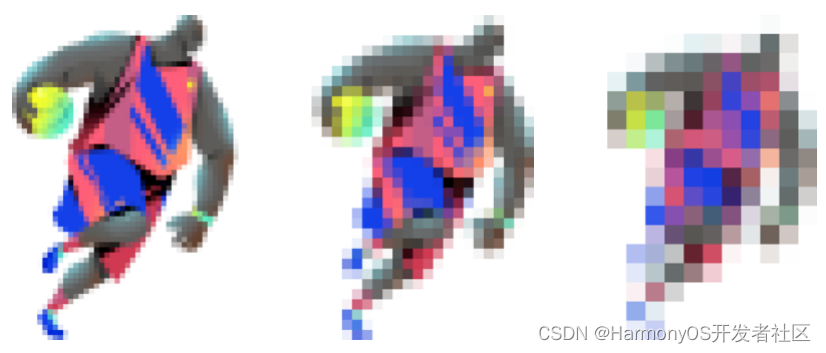图6 马赛克强弱控制

1. 首先我们需获取ArkUI开发框架的image能力，该能力提供了图片开发的基本接口。

`import image from "@ohos.multimedia.image" `

`let bufferData = new ArrayBuffer(bitmap.getPixelBytesNumber()); `

`await bitmap.readPixelsToBuffer(bufferData); `

`for (let index = 0; index < dataArray.length; index += 4) { `

`const r = dataArray[index]; `

`const g = dataArray[index+1]; `

`const b = dataArray[index+2]; `

`const a = dataArray[index+3]; `

`} `

3. 根据自定义的单个小方格的Width和Height，将整个图片分成若干小方格。

`//横排的正方形个数 `

`var x_index = Math.floor(targetWidth / realPixel_W); `

`//纵排的正方形个数 `

`var y_index = Math.floor(targetHeight / realPixel_H); `

4. 获取每个小方格左上角的最大坐标及右下角的最小坐标，以确定小方格的区域。并根据每个小方格内的所有像素点数据统一该区域的像素，统一方式可以是取该区域内像素点的平均值，或者随机选取一个像素。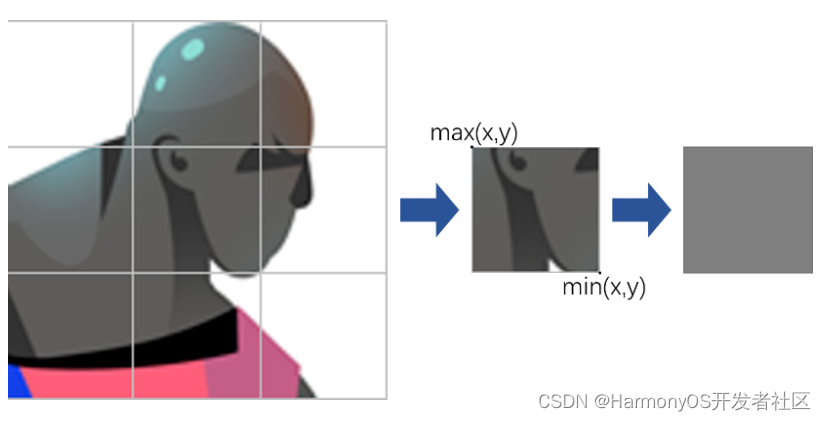`for (let ch = 0; ch < y_index; ch++) { `

`for (let cw = 0; cw < x_index; cw++) { `

`let max_x = (cw + 1) * realPixel_W > targetWidth ? targetWidth : (cw + 1) * realPixel_W; `

`let max_y = (ch + 1) * realPixel_H > targetHeight ? targetHeight : (ch + 1) * realPixel_H; `

`let min_x = cw * realPixel_W; `

`let min_y = ch * realPixel_H; `

`//取左上角的像素值 `

`let center_p = inPixels[min_y+1][min_x+1]; `

`//设置该正方形里的像素统一 `

`for (let zh = min_y; zh < max_y; zh++) { `

`for (let zw = min_x; zw < max_x; zw++) { `

`inPixels[zh][zw] = center_p; `

`} `

`} `

`} `

`} `

5. 通过writeBufferToPixels接口，将统一的像素点数据缓存到ArrayBuffer中，并写入PixelMap，由此得到整张马赛克处理的图片。

`writeBufferToPixels(src: ArrayBuffer): Promise `

1. 给图片添加Touch事件，获取手指的运动轨迹。参考代码如下：

`Image(this._mCropPixelMap.pixelMap) `

`.width(300) `

`.height(300) `

`.margin(10) `

`.objectFit(ImageFit.Contain) `

`.onTouch(event => { `

`let array: TouchObject[] = event.changedTouches; `

`for (let i = 0;i < array.length; i++) { `

`//触摸的x y坐标 `

`let centX = array[i].x; `

`let centY = array[i].y; `

`} `

`}); `

2. 根据运动轨迹，以触摸点的坐标（x，y）为中心，根据自定义小方格的大小，动态确认马赛克区域的位置。参考代码如下：

`//获取到左上角的坐标 `

`let offMinX = Math.floor(centerX - pixel / 2); `

`let offMinY = Math.floor(centerY - pixel / 2); `

`//右下角的坐标 `

`let offMaxX = Math.floor(centerX + pixel / 2); `

`let offMaxY = Math.floor(centerY + pixel / 2); `

`offMinX = offMinX < 0 ? 0 : offMinX; `

`offMinY = offMinY < 0 ? 0 : offMinY; `

`offMaxX = offMaxX > targetWidth ? targetWidth : offMaxX; `

`offMaxY = offMaxY > targetHeight ? targetHeight : offMaxY; `

3. 统一马赛克区域的所有的像素点值。

`//取左上角的像素值 `

`let center_p = PixelExampleUtils.inPixels[offMaxY+1][offMaxX+1]; `

`//设置该正方形里的像素统一 `

`for (let zh = offMinY; zh < offMaxY; zh++) { `

`for (let zw = offMinX; zw < offMaxX; zw++) { `

`PixelExampleUtils.inPixels[zh][zw] = center_p; `

`changeArray[zh][zw] = center_p; `

`} `

`} `

4. 最后将更改的像素点写入图片中，即可得到手指滑动轨迹的马赛克图片。